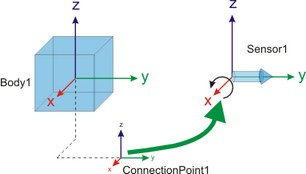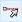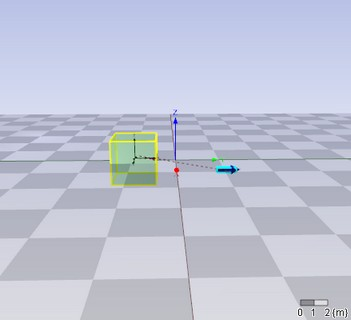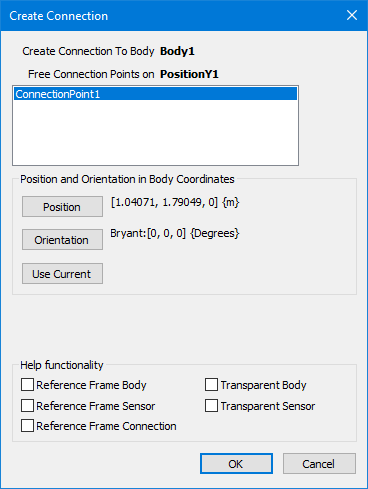﻿ 20-sim webhelp > Toolboxes > 3D Mechanics Toolbox > Library > Sensors

# Sensors

Sensors are components that indicate the position or velocity of bodies as output signals.

## Connecting Sensors

Sensors have to be connected with bodies, just like joints.The position of the sensor is indicated by its connection point. The connection point is shown automatically when you make a connection between a body and a sensor. First change to Connection mode (), then click the body and then click the sensor.The Create Connection dialog will pop to ask you for the location of the connection point. In the connection dialog you can set the offset (both the position as well as rotation) from the body reference frame to the connection point.## Sensor Types

Various sensor types are available in the library.

## Position/Orientation

 sensor output signal Position-X A signal with the x-position of the body. Position-Y A signal with the y-position of the body. Position-Z A signal with the z-position of the body. Position (3x1) A signal (size 3) with the x-, y- and z-position of the body. H-matrix (4x4) A 4x4 signal with the H-matrix. Rotation (3x3) A 3x3 signal with the rotation-matrix.

## Velocity

 sensor output signal Velocity-X A signal with the x-velocity of the body. Velocity-Y A signal with the y-velocity of the body. Velocity-Z A signal with the z-velocity of the body. Velocity (3x1) A signal (size 3) with the x-, y- and z-velocity of the body. Omega-X A signal with the rotational velocity in x-direction of the body. Omega-Y A signal with the rotational velocity in y-direction of the body. Omega-Z A signal with the rotational velocity in z-direction of the body. Omega (3x1) A signal (size 3) with all three rotational velocities.

## Acceleration

 sensor output signal Acceleration-X A signal with the x-acceleration of the body. Acceleration-Y A signal with the y-acceleration of the body. Acceleration-Z A signal with the z-acceleration of the body. Acceleration (3x1) A signal (size 3) with the x-, y- and z-acceleration of the body. Rotational Acceleration-X A signal with the rotational acceleration in x-direction of the body. Rotational Acceleration-Y A signal with the rotational acceleration in y-direction of the body. Rotational Acceleration-Z A signal with the rotational acceleration in z-direction of the body. Rotational Acceleration (3x1) A signal (size 3) with all three rotational accelerations.

## Jacobian Matrix

This yields the Jacobian Matrix of the connected mass.